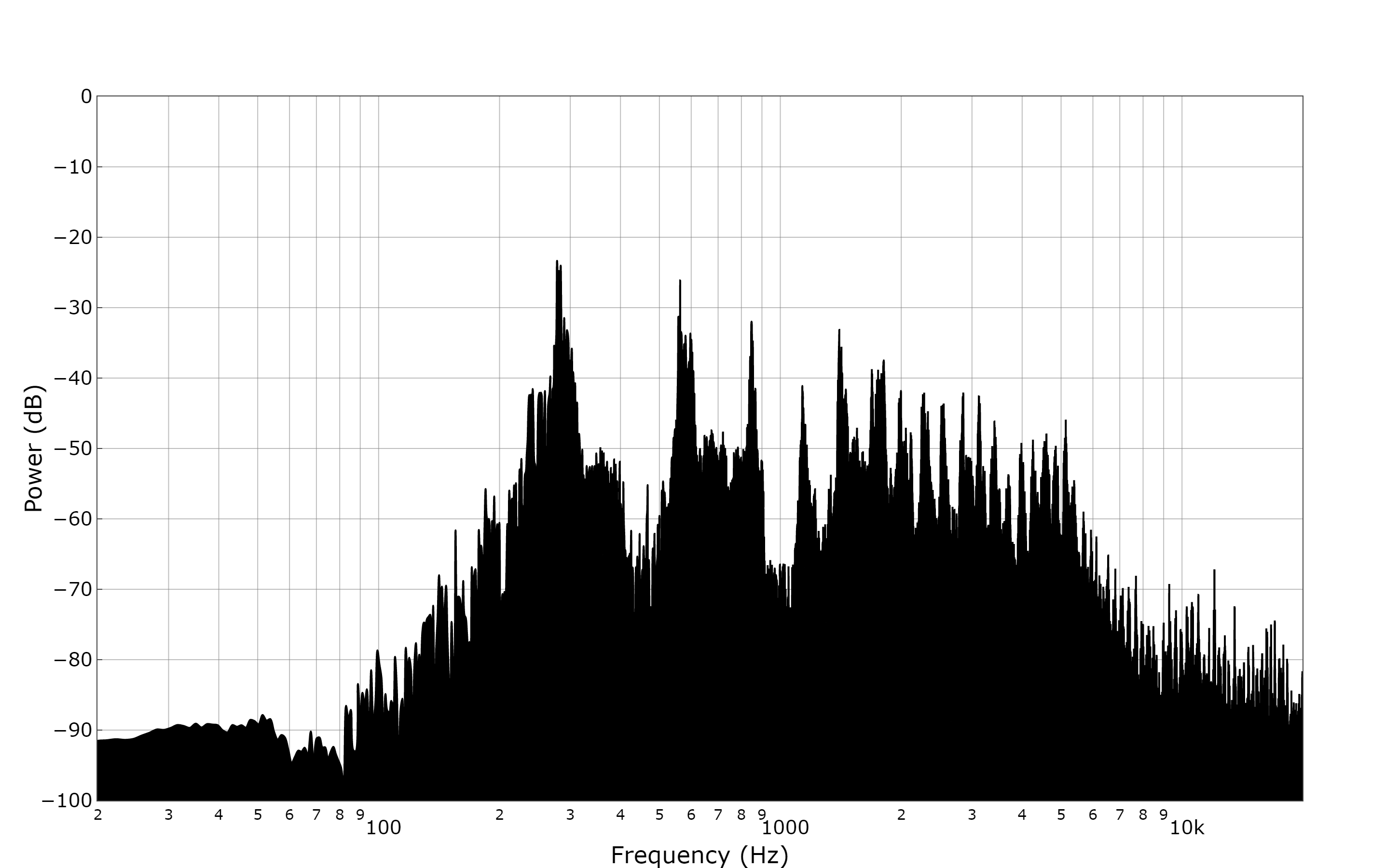# FFT From A WAV FileThis example builds upon the script used in the time domain example. R can be used to carry out an FFT of the wav file and plot it in the same way as the octave band example. See the script below (courtesy of Sam Carcagno) that will carry out an FFT.

```n <- length(s1)
fft <- fft(s1)

nUniquePts <- ceiling((n+1)/2)
fft <- fft[1:nUniquePts] #select just the first half since the second half
# is a mirror image of the first
fft <- abs(fft) #take the absolute value, or the magnitude

fft <- fft / n #scale by the number of points so that
# the magnitude does not depend on the length
# of the signal or on its sampling frequency
fft <- fft^2 # square it to get the power

# multiply by two (see technical document for details)
# odd nfft excludes Nyquist point
if (n %% 2 > 0){
fft[2:length(fft)] <- fft[2:length(fft)]*2 # we've got odd number of points fft
} else {
fft[2: (length(fft) -1)] <- fft[2: (length(fft) -1)]*2 # we've got even number of points fft
}

freqArray <- (0:(nUniquePts-1)) * ([email protected] / n) # create the frequency array```

Now we can add this back in to the data frame used previously using:

`mydata <- data.frame(freqArray,10*log10(fft))`

Notice the use of the 10*log10(fft) to compute the power in dB.

Now we need to make some edits to the plot. Start by adding the lines below after “add_trace”:

```fill = 'tozerox',
fillcolor = 'rgb(0, 0, 0)',```

This will fill in the area under the trace in black. Adjust width to your liking.

To set xaxis range to 20 Hz – 20 kHz use:

```range = c(1.3, 4.3),
type = "log"```### References

http://samcarcagno.altervista.org/blog/basic-sound-processing-r/?doing_wp_cron=1534332338.3809800148010253906250